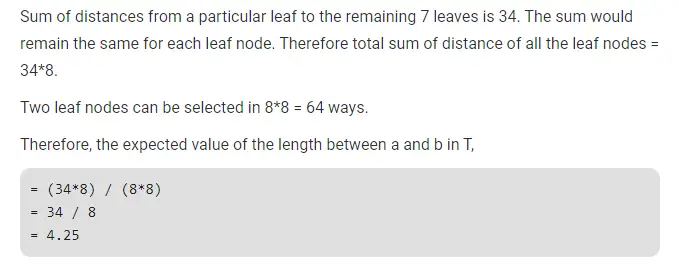# Gate CS-2019 Question Paper With Solutions

Q. 55 Let T be a full binary tree with 8 leaves. (A full binary tree has every level full.) Suppose two leaves a and b of T are chosen uniformly and independently at random. The expected value of the distance between a and b in T (i.e., the number of edges in the unique path between a and b) is (rounded off to 2 decimal places) ___________ .

Note: This was Numerical Type question.

(A) 5.71 to 5.73

(B) 4.85 to 4.86

(C) 2.71 to 2.73

(D) 4.24 to 4.26